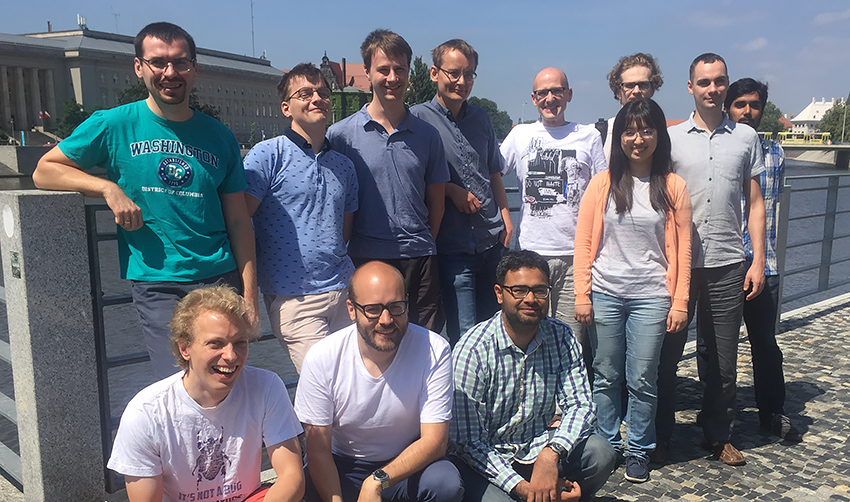Our research focuses on discrete optimization problems arising in network design, graph theory, scheduling, logistic and planning. We design efficient algorithms with provable guarantees on the quality of constructed solutions. We apply and develop methods from different branches of mathematics and theoretical computer science, such as mathematical programming, combinatorics, and algorithm theory.

Our areas of expertise include:

• approximation algorithms for NP-hard graph problems
• online algorithms for network problems
• algorithms for traveling salesperson problems
• structure of graph matchings
• online algorithms for scheduling problems
• rounding linear programming relaxations
• algorithmic game theory